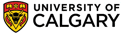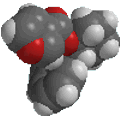Chapter 13: Spectroscopy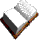Coupling in H-NMR

So far the H-NMR spectra that we have looked at have all had different types of protons that are seen as singlets in the spectra.  This is not the normal case.... spectra usually have peaks that appear as groups of peaks due to coupling with neighbouring protons, for example, see the spectra of 1,1-dichloroethane shown below.Before we look at the coupling, lets review the assignment of the peaks first:
• δ = 5.9 ppm, integration = 1H deshielded : agrees with the -CHCl2 unit
• δ = 2.1 ppm, integration = 3H : agrees with -CH3 unit
Now, what about the coupling patterns ?

Coupling arises because the magnetic field of vicinal (adjacent) protons influences the field that the proton experiences.
 To understand the implications of this we should first consider the effect the -CH group has on the adjacent -CH3.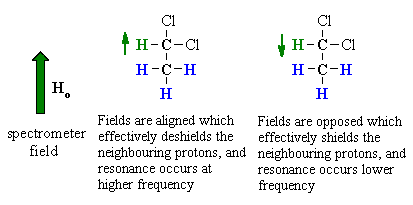The methine -CH can adopt two alignments with respect to the applied field. As a result, the signal for the adjacent methyl -CH3 is split in two lines, of equal intensity, a doublet. Now consider the effect of the -CH3 group has on the adjacent -CH .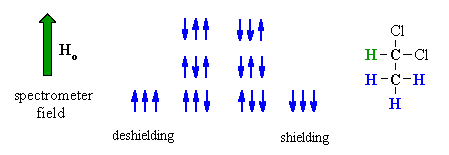The methyl -CH3 protons give rise to 8 possible combinations with respect to the applied field. However, some combinations are equivalent and there are four magnetically different effects. As a result, the signal for the adjacent  methine -CH is split into four lines, of intensity ratio 1:3:3:1, a quartet.

• The proximity of  "n" equivalent H on neighbouring carbon atoms, causes the signals to be split into "n+1" lines.
• This is known as the multiplicity or splitting or coupling pattern of each signal.
• Equivalent protons (or those with the same chemical shift) do not show coupling to each other.
• If the neighbours are not all equivalent, more complex patterns arise (this is because of different J values, see below).
• When looking at H-NMR, the most common couplings that are observed are those between H atoms on neighbouring C atoms (i.e. vicinal coupling, Ha-C-C-Hb where 3 bonds are involved)
• To a first approximation, protons on adjacent sp3 C tend to behave as if they are equivalent (for example, the H-NMR of 1-bromopropane)
Now we can do more a complete analysis, including the application of the "n+1" rule to 1,1-dichloroethane:
• δ = 5.9 ppm, quartet, integration = 1H, deshielded : agrees with the -CHCl2 unit next to a -CH3 unit (n = 3, so n + 1 = 4 lines)
• δ = 2.1 ppm, doublet, integration = 3H : agrees with -CH3 unit, next to a -CH- (n = 1, so n + 1 = 2 lines)
Coupling Constant, J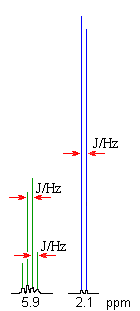The coupling constant, J (usually in frequency units, Hz) is a measure of the interaction between a pair of protons.  In a vicinal system of the general type, Ha-C-C-Hb then the coupling of Ha with Hb, Jab,  MUST BE EQUAL to the coupling of Hb with Ha, Jba, therefore Jab = Jba.  The implications are that the spacing between the lines in the coupling patterns are the same as can be seen in the coupling patterns from the H-NMR spectra of 1,1-dichloroethane (see left).

Typical Coupling Constants

So far we have emphasised vicinal coupling of H atoms on adjacent sp3 C atoms. This coupling constant is typically about 6-8 Hz. Coupling is controlled by geometry and the orbitals involved between the coupling nuclei and therefore other types of systems have slightly different coupling constants as shown below:Notes:

• H atoms on a CH2 of a alkene don't have to be equivalent (depending on what substituents are on the other end of the alkene). As a result they can couple to each other. This is a 2 bond coupling.
• Coupling between H atoms that are more than 3 bonds is also possible and is known as "long range coupling".
• In general, the more bonds involved between the H that are coupling, the smaller the J value.
• Long range coupling is more common with rigid systems including the pi bond systems of alkenes and substituted benzenes.
• The presence of different couplings (i.e. different J values) tends to lead to "complex coupling" (more on that later).

Pascal's Triangle

 The relative intensitites of the lines in a coupling pattern is given by a binomial expansion or more conviently by Pascal's triangle.  To derive Pascal's triangle, start at the apex, and generate each lower row by creating each number by adding the two numbers above and to either side in the row above together. The first six rows are shown to the right.  So for H-NMR a proton with zero neighbours, n = 0,  appears as a single line, a proton with one neighbours, n =1 as two lines of equal intensity, a proton with two neighbours, n = 2, as three lines of intensities 1 : 2 : 1,   etc.QUESTIONS
• What would the multiplicity and the relative intensitites be for the secondary H in propane ? ANSWER
• What is the multiplicity for the tertiary H in 2-methylpropane ? ANSWER

Summary

• Individual resonances are split due to coupling with "n" equivalent adjacent protons
• Number of lines in coupling pattern, L = n + 1© Dr. Ian Hunt, Department of Chemistry